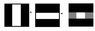# Fourier transform sum of two images

• I
• BobP

#### BobP

The FT decomposes images into its individual frequency components
In its absolute crudest form, would the sum of these two images (R) give the L image?

#### Attachments

•FT.png
304 bytes · Views: 405
The sum should also have the vertical grey arm. But I just don't see how those pictures connect to the subject of FT.

The sum should also have the vertical grey arm. But I just don't see how those pictures connect to the subject of FT.
I thought the FT was about decomposing images into different frequencies. So I was showing the inverse of what I thought the FT and asking if it was correct as it was easier for me to show it this way.
Is what I showed not related?

You can use those pictures as an analogy to an FT, because FT is about summing functions of the form ##e^{ik_xx}## (for 1D) and ##e^{ik_xx}e^{ik_yy}## (for 2D) having certain amplitude distribution.

•BobP
What are R and L?

I'm not exactly sure what you are asking,. But, the Fourier transform is a linear map. So F{f(x)+g(x)} = F{f(x)} +F{g(x)}.

What are R and L?
Right and left (referrring to the images)

You can use those pictures as an analogy to an FT, because FT is about summing functions of the form ##e^{ik_xx}## (for 1D) and ##e^{ik_xx}e^{ik_yy}## (for 2D) having certain amplitude distribution.
Ok, in addition then which of the two examples shown correctly represents the transformation between the spatial domain (right) and domain (left)

I am only learning the FT to gain a very crude understanding of how image reconstruction is done. As I do not have a physics background the course organiers are not teaching the maths

#### Attachments

Ok, in addition then which of the two examples shown correctly represents the transformation between the spatial domain (right) and domain (left)
To me, the bottom picture seems to correspond to a pair of Fourier transform conjugates (can you see why?).

To me, the bottom picture seems to correspond to a pair of Fourier transform conjugates (can you see why?).
Presumably because it is symmetrical about the centre of k-space.
But as the image only displays a single frequency I wasn't sure if I only needed to display two conugate pairs or lots

By your answer am I correct in assuming you think the bottom image is correct then?

Presumably because it is symmetrical about the centre of k-space.
But as the image only displays a single frequency I wasn't sure if I only needed to display two conugate pairs or lots

By your answer am I correct in assuming you think the bottom image is correct then?
A way of justifying the bottom images is to do the math. The right picture of the bottom pair looks like it being composed of three delta functions. Mathematically, it reads
$$f(x,y) = \delta(y) (\delta(x+a) + \delta(x) + \delta(x-a))$$
Now Fourier transform ##f(x,y)## and see if you will get something that resembles the left picture.

A way of justifying the bottom images is to do the math. The right picture of the bottom pair looks like it being composed of three delta functions. Mathematically, it reads
$$f(x,y) = \delta(y) (\delta(x+a) + \delta(x) + \delta(x-a))$$
Now Fourier transform ##f(x,y)## and see if you will get something that resembles the left picture.
Hi. Like I said I don't know any of the maths so I am learning everything conceptually.
So I don't really know what you mean by 3 delta functions :(

Like I said I don't know any of the maths so I am learning everything conceptually.
FT is one subject of math, there is no other way to learn FT except by learning the maths.
Delta functions is a mathematical object usually used to represent a zero-dimensional point. In reality obviously there is no such object, however if a pinhole is much smaller compared to the wavelength of light illuminating it (in the case of the diffraction of light), it can be modeled as a delta function.

FT is one subject of math, there is no other way to learn FT except by learning the maths.
Delta functions is a mathematical object usually used to represent a zero-dimensional point. In reality obviously there is no such object, however if a pinhole is much smaller compared to the wavelength of light illuminating it (in the case of the diffraction of light), it can be modeled as a delta function.
I see. well thank you for the confirmation but as I have a very limited knowledge of calculus it seems like it'll be a long time before I can prove why the bottom image is correct.

Thanks again for your help though :)

The bottom image looks like the correct amplitude or power spectrum of the original image, which is based on the magnitude of the complex Fourier transform. However, you won't be able to reconstruct an image without a corresponding phase spectrum that comes from the argument of the same transform.

The bottom image looks like the correct amplitude or power spectrum of the original image, which is based on the magnitude of the complex Fourier transform. However, you won't be able to reconstruct an image without a corresponding phase spectrum that comes from the argument of the same transform.
thanks :)